Next:Characterizations of Generalized EntropiesUp:Entropy-Type Measures

Unified (r,s)-Entropy for Continuous Probability Distributions

In this section we extend the notion of generalized entropies to the continuous case, and examine some properties of the resulting unified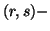entropy function.

Let X be an absolutely continuous random variable, that is, a random variable having a probability density function p(x). The unifiedentropy of X is defined as follows: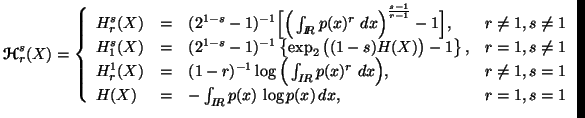(3.14)

provided the integrals exist, where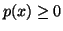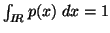.

The contrast between continuous and discrete distribution is worth emphasising:

(i) The entropy measure of continuous distribution need not exist.
(ii) When it does exist, there is nothing to ensure that is positive because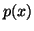can exceed unity. We consider the following examples:
Example 3.1. Let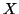be a random variable with probability density function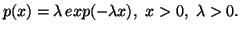Then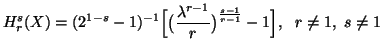with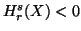when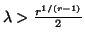. (iii) The unifiedentropy are not limits of the unifiedentropy of the discrete case. This we shall verify in the following example.

Example 3.2. Letbe a discrete random variable taking the values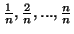with equal probabilities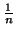. Then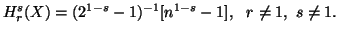As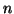increases, the distribution ofconverges to a continuous uniform distribution in (0,1). If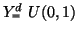, we have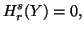however,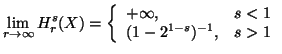(iv) The unifiedentropy is not invariant with respect to a change of variables. We illustrate this point with the following example:

Example 3.3. We consider a function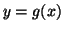, where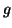is a stricly increasing function of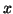. Since the mapping fromto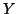is one to one, we have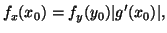where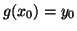. Therefore,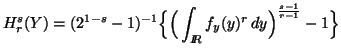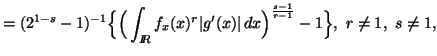which is different from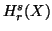unlessbe the identity function.

These important differences between discrete and continuous cases are a warning that the results for the discrete distributions cannot be translated to continuous case without independent verification. Fortunately, some of the significant concepts rely upon differences between entropies and for these the difficulties disappear.

21-06-2001
Inder Jeet Taneja
Departamento de Matemática - UFSC
88.040-900 Florianópolis, SC - Brazil